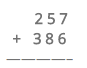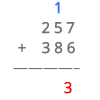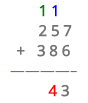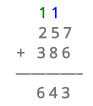Smartick is an online platform for children to master math in only 15 minutes a day

Nov18

In today’s post, we are going to learn about addition with regrouping.

Addition with regrouping consists of addition problems where the answer to the calculation is 10 or a larger number. For example, 6 + 7 = 13 is a regrouping addition problem.

The steps to solve regrouping addition problems are the following:

• If the column being added doesn’t have a carry-over (or if the number is lower than 10), write the answer under the column.
• If the column being added has a carry-over (or if the number is equal to or greater than 10), write the 1s-place numbers down under the bar and take note of the 10s-place number in the next column to add them later.

Let me show you an example of adding with regrouping. Try it with me!!Start by adding the 1s-place columns:

### 7 + 6 = 13

This number is greater than 10, so that means you need to write 3 under the 1s-place column and the 1 (the carry-over) above the next column.Now, you just add the next column, and don’t forget about the carry-over:

### 1 + 5 + 8 = 14

This number also has a carry-over. Write the 4 down in the column of the 10s-place and bring the 1 up to the next column.Now, we just need to add the last column:

### 1 + 2 + 3 = 6

The only thing left is to write that number underneath in its appropriate column:So the final answer to this addition problem is 643. Did you get it right?

Do you have any questions? You can use our comments to get some help.

I hope you liked learning how to add with regrouping and I also hope that you understand it well!

If you want to keep learning more elementary math, try Smartick for free.